# The Top 100+ Sines of Wolfram|Alpha

August 24, 2012 —Comments Off

While Wolfram|Alpha can do many sorts of computations, mathematical calculations are one of its particular specialties. In fact, using the power of Mathematica‘s computational capabilities under the hood, Wolfram|Alpha can do many things, ranging from the very simple to the fiendishly complicated, with mathematical functions.

To highlight what we mean by “many” (and we do indeed mean it), let’s take the unassuming sine function as an example. Here is a list that started out as 93 possibilities of what Wolfram|Alpha can do with sine (one for each day of summer), but ended up including a number of bonus inputs above and beyond that which we couldn’t resist throwing in.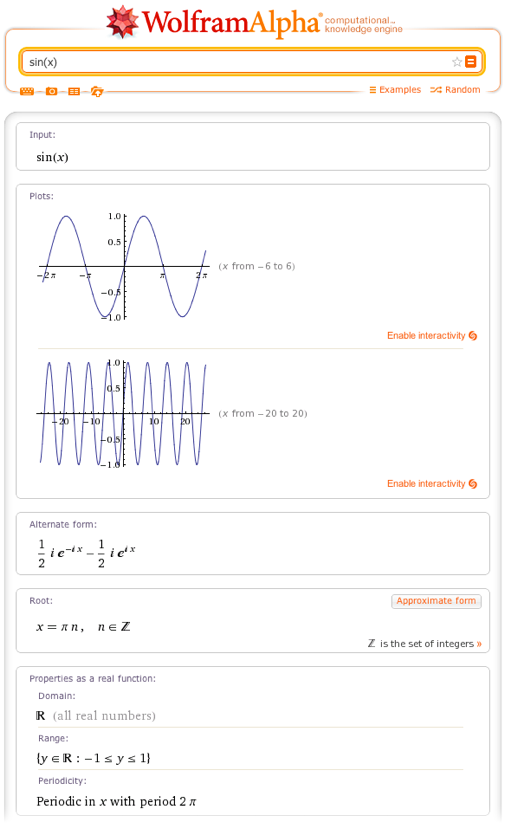For those who know about such things, most of the results above apply to real values of the argument x. If on the other hand you use a different form of the argument such as sin(z) or sin(x + i y), Wolfram|Alpha will prefer an interpretation assuming complex variables, and so give a different set of plots and results: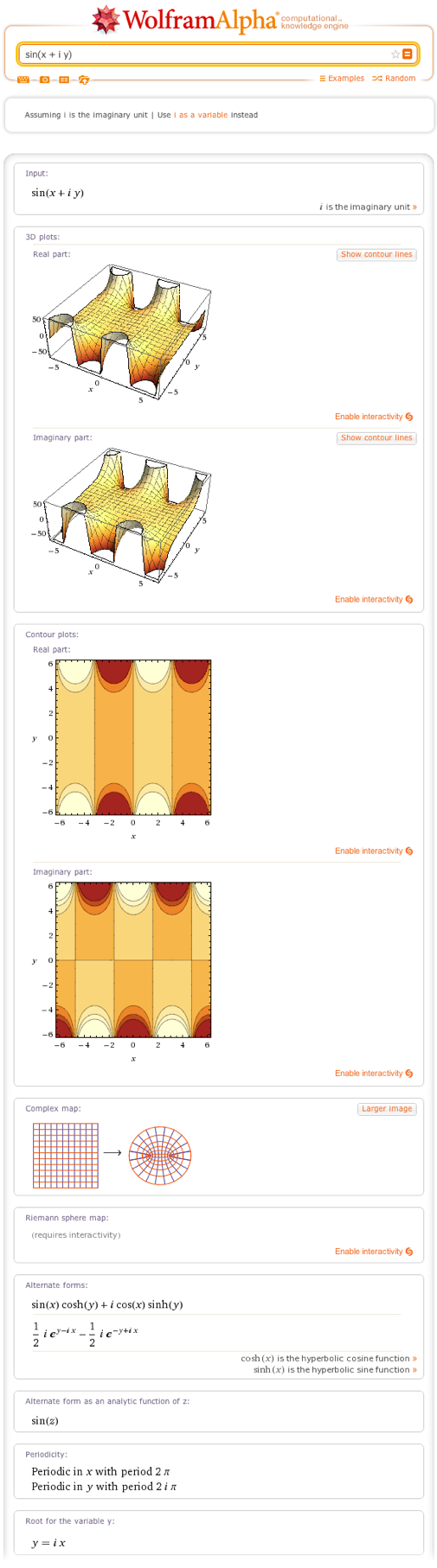Of course, one can request each of the above computational results individually, for example, global maxima sin(x). One can also generate a multitude of plots by specifically asking for them, including: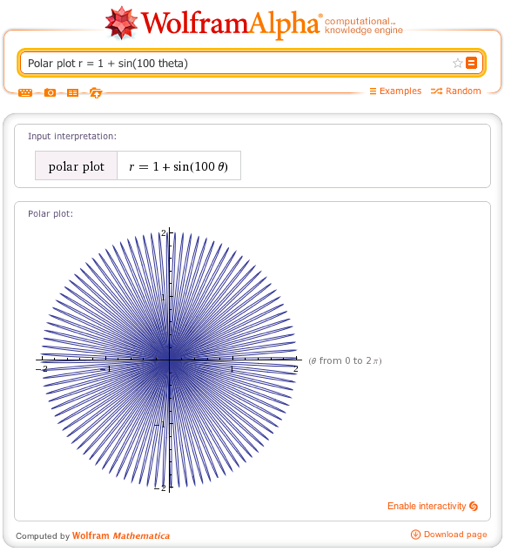One can also easily plot more complicated expressions of the sine function, such as:

And even many functions using literal Mathematica syntax, such as:

One can calculate special values of the sine function, such as sin(pi/88). Conversely, given the resulting value 0.0356923338389804557600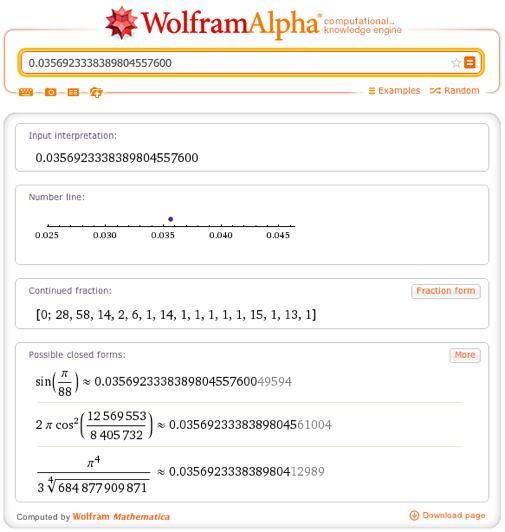…one can recognize it as a value of the sine function at x = pi/88 (the first of the three possible closed forms returned as shown above). So motivated, one might consider asking for a list of all special values at once using the input special values of sin(x). A particularly beautiful example in this class is function expand sin(pi/17), which shows the classic result that Gauss found on his 17th birthday.

One can ask for special forms of values and properties of values directly:

Find periods:

And find minima and maxima of functions containing sine:

One can draw 2D and 3D Lissajous figures: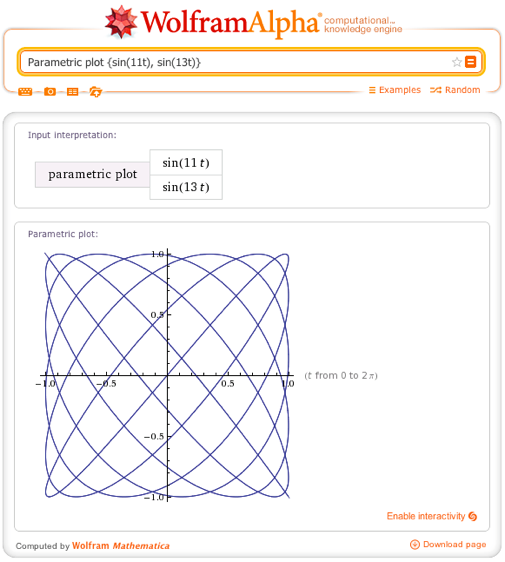And get their curvatures:

One can ask for inflection points:

Arc lengths: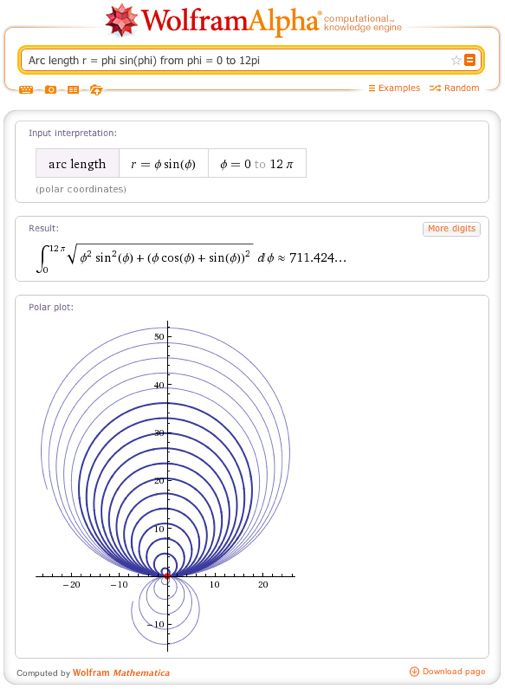Corners:

And periodicity:

There are a multitude of mathematical formulas that can be requested. For concrete cases, consider:

As well as general formulas:Wolfram|Alpha also knows about many specific kinds of representations:

One can ask for the relation of the sine functions with other functions:

Including its relation with the inverse function and other functions expressed in terms of sine: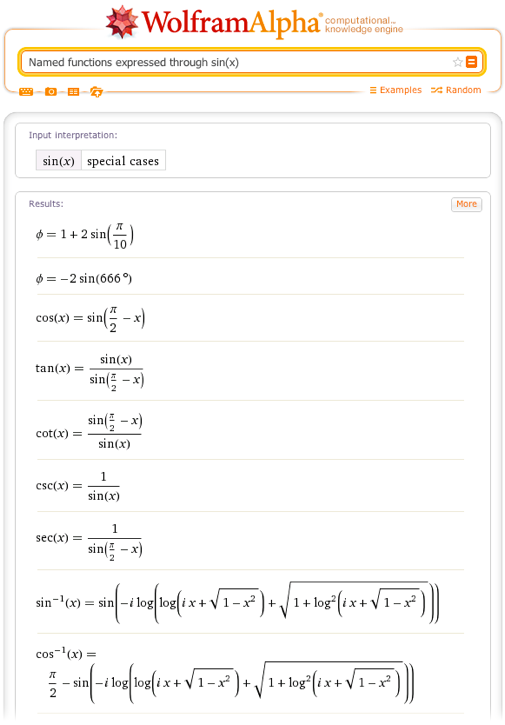One can solve equations containing the sine functions:

Prove trigonometric identities involving the sine function:

Find discontinuities and poles of expressions containing sine:

Differentiate the sine function:

Carry out sums:

And, of course, integrate functions of sine: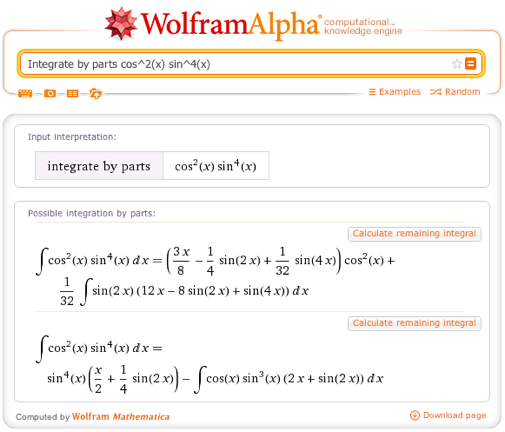One can get sample lists of sums, products, and integrals:

Integral transforms:

And find limits: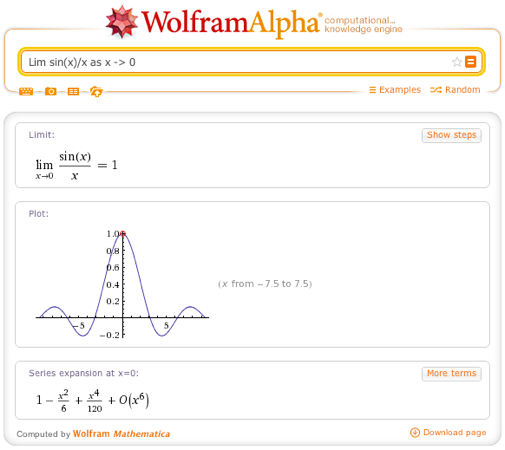One can expand the sine function in a series:

Sum the Taylor series to finite order in closed form:

Or get a Pade approximation of the sine function:

One can simplify more complicated expressions involving sine: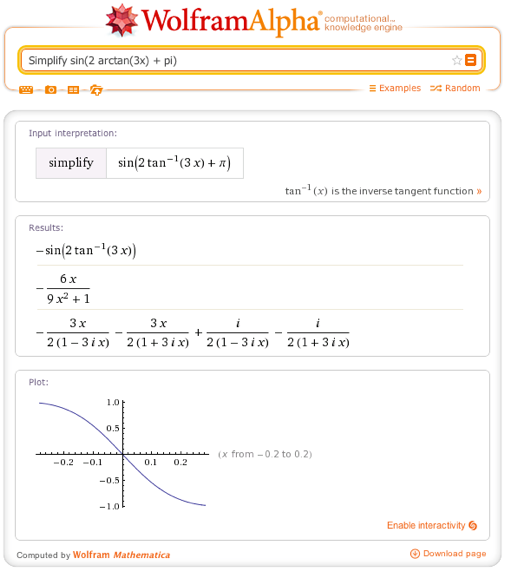And verify identities:

As well as expand a function into a Fourier sin series:

The sine function has many relatives and generalizations, including but not limited to:

One can use the sine function in probability calculations, for example, to find the expectation for sin(x) assuming x is distributed normally: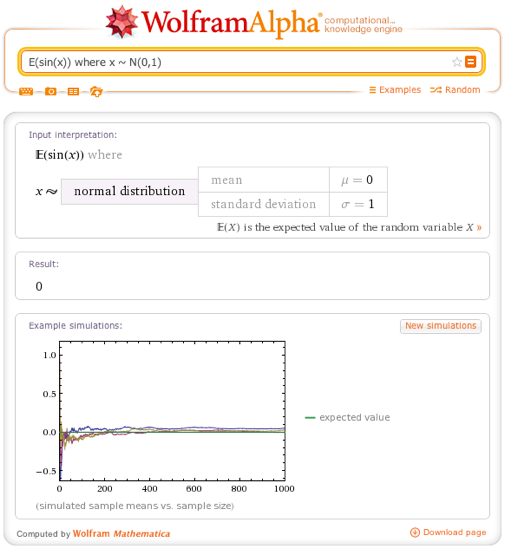One can solve differential equations involving the sine function:

Calculate Wronskians involving the sine functions:

And find the differential equation obeyed by an expression containing the sine function:

One can use the sine law and the spherical law of sines: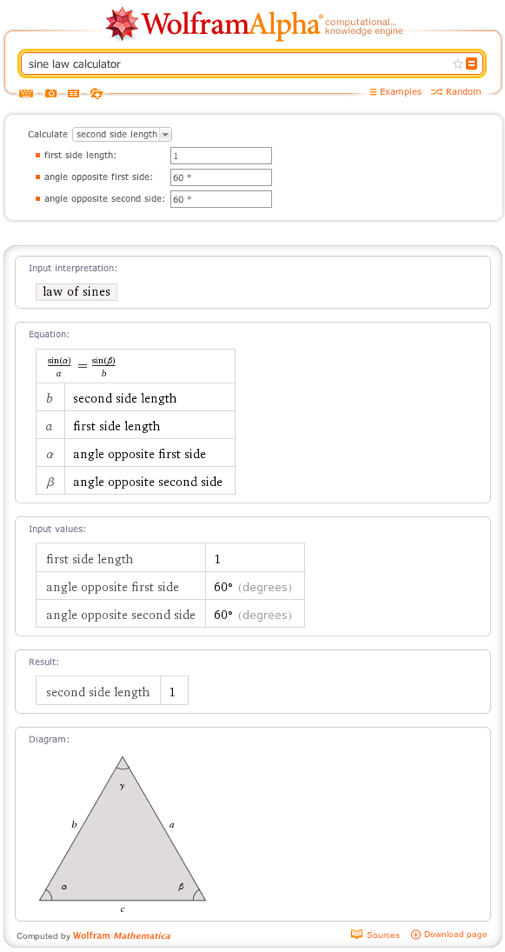Express and approximate the value of pi through values of the sine function:

And request an interactive version of the sine function:

One can even do computations on URL-referenced objects on the internet, for example, providing analysis of an image of a sine function taken from The Wolfram Functions Site: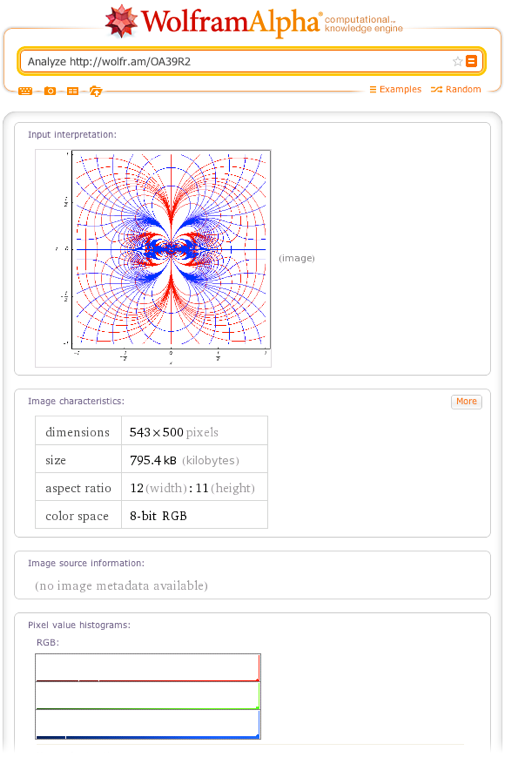Last, not least, one can “play” the sine function:

We could go on (and on, and on), but that almost certainly suffices (“many” indeed). So as many of you are going back to school after what we hope was an enjoyable summer, please keep Wolfram|Alpha and its powerful collection of functionality in mind as you resume your studies. While we realize not everyone will enjoy getting back into the academic term as much as they did the allegedly halcyon days of summer, we hope there are at least a few of you out there who will have more fun learning with the help of Wolfram|Alpha, now that you know about it, than you did during your long, boring summer. 🙂

Great post! Really fun ideas with plain ole sine!

Posted by Mimi August 24, 2012 at 2:05 pm

No integral equation with sine??

Posted by Fred Holm August 26, 2012 at 10:12 am

Commands are rather standard. Still the list is for an analysis of a single function.

The point, which is not clear is “And verify identities …”. It seems that standard “simplify” is more universal and definitely more useful in a routine applications.

Posted by sv August 31, 2012 at 11:45 am

cool..

Posted by Pedro August 12, 2013 at 12:49 pm

A lot of good ideas. Much appreciated. One that I’ve used several times is using a table to plot several curves in one graph. However, I have a question. How can I label the curves?

In the absence of instructions on my part, the plot shows on the right a colored line segment for each curve next to the individual equation written out in detail. While this does tell me which curve is which, it is not very useful for a complicated equation and I would like to label each color with, say, the values of the variable I use to step through the table. Is there a way to do that? Thanks.

Posted by Stuart McAlpine April 5, 2014 at 2:28 pm

Are these from a cache-based database or does Wolf use a hybrid system to fetch results for an equation :S

Posted by Mr Bitcoin November 21, 2014 at 5:59 pm